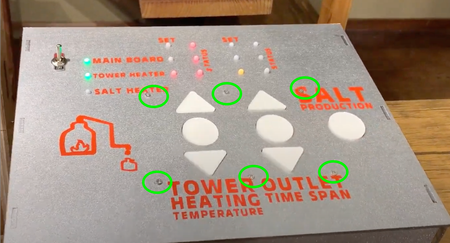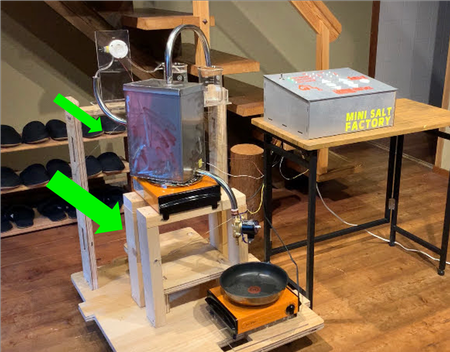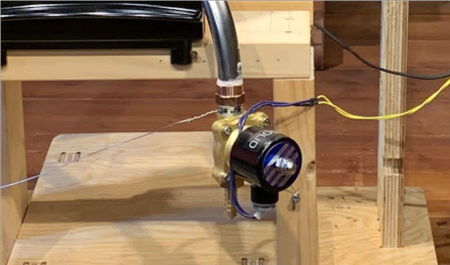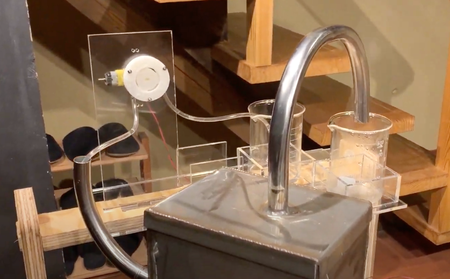# Final project - programming & integration¶

## Program¶

The code of switch scanning process, setting LED pattern, controling SSR/solenoid valve are all based on the those stated in the electronics session. The whole program is about integrating them.

A very important point I learned from Yamamoto-san is to not have multiple `delay()` in one program so they wouldn’t add up.

For example, if I have `delay(100)` in the session for switch group1 and another `delay(100)` in the session for switch group2, they would add up, and the whole loop will delay 200 micro seconds in total.

In this program, there is no `delay` so the delay for each loop would be 0, thus the time for each loop would be the time it takes to proceed the whole loop.

And when I want to count time, I set up a counter variable.

For example if I want to turn on solenoid valve after 10000 loop cycles, I used a variable `counter_sol_OFF`.

In each loop, I used `counter_sol_OFF ++` to add 1 to the variable. And once it is over 10000, it `digitalWrite(solenoidPin, HIGH);`.

`````` counter_sol_OFF ++ ;
matrix =0;
matrix =0;
matrix =0;
if( counter_sol_OFF > 10000 ){
counter_sol_ON = 0;
counter_sol_OFF = 0;
sol_state = 1;
digitalWrite(solenoidPin, HIGH);
``````

This is the entire code that is constructed by me, with the revision of Yamamoto-san.

``````//
//
//
//  2020.7.20 16:45
//

int matrix = {
{0,0,0,0},
{0,0,0,0},
{0,0,0,0},
{0,0,0,0}
};
const int analogSG1 = A0;
const int analogSG2 = A1;
const int analogSG3 = A2;
const int analogTHER = A3;
int relay2Pin = 3; //set pin 3 for relay2 (heater2 = pan) output
int relay1Pin = 2; // set pin 2 for relay1 (heater1 = tower) output
int motorPin = 13;
int solenoidPin = 12;
int valSG1=0; //value of switch group 1
int oldvalSG1=0; //old value of switch group 1
int LEDstateSG1=0; //LED state of switch group 1
int valSG2=0; //value of switch group 2
int oldvalSG2=0; //old value of switch group 2
int LEDstateSG2=0; //LED state of switch group 2
int valSG3=0; //value of switch group 3
int oldvalSG3=0; //old value of switch group 3
const float R4 = 100000; //R4 is R4 in the circuit, the resistor next to thermistor
int mainonoff1 = 0; // switch controlled onoff of heater1
int onoffstate1 = 0; //actual onoff state of heater1
int mainonoff2 = 0; // switch controlled onoff of motor
int mainonoff3 = 0; // switch controlled onoff of solenoid
int activebutton = 0; //indicates which button is pressed
int counter_motor = 0;
int counter_sol_ON = 0;
int counter_sol_OFF = 0;
int counter_sol_lmt = 0;
int counter_serial = 0;
int counter_sumTActual = 0;
int counter_LED = 0;
int keySG1, keySG2, keySG3, old_keySG1, old_keySG2, old_keySG3;
int col;

int sol_state; // solenoid ON/OFF state

float ave_TActual, sum_TActual;

void setup() {
Serial.begin( 9600 );
pinMode(relay1Pin, OUTPUT);
pinMode(relay2Pin, OUTPUT);
pinMode(motorPin, OUTPUT);
pinMode(solenoidPin, OUTPUT);
//Set up pins for LED matrix
for (int i=5;i<=11;i++)
{
pinMode(i, OUTPUT);
digitalWrite(i, LOW);
}
digitalWrite(motorPin, LOW);
digitalWrite(solenoidPin, LOW);
}
void loop()
{
// thermistor to read TActual: the current temperature of tower
float valTher,V,R,TActual;
float TSet; //Tset is the set temperature for heater1
V = 5*valTher/1024;
R = (5-V)*R4/V;
float y = 4076.6/(log(R)+2.11);
TActual=y-273;
sum_TActual = sum_TActual + TActual;
if (counter_sumTActual > 500) {
ave_TActual = sum_TActual/500;
counter_sumTActual = 0;
sum_TActual = 0;
Serial.println (ave_TActual);
}
counter_sumTActual = counter_sumTActual + 1;

//*********************************************
// Switch scan process
//*********************************************

// *** Read switch group1 ***

if (valSG1 <250) {
keySG1 = 1;
} else if ( valSG1 < 600 ) {
keySG1 = 2;
} else if ( valSG1 < 800 ) {
keySG1 = 3;
} else {
keySG1 = 0;
}

// *** Ckeck Switch push **

if(keySG1 != old_keySG1 ) {
if ( keySG1 == 1 ) {
LEDstateSG1 = (LEDstateSG1+1) % 3;
} else if ( keySG1 == 3 ){ //if sw3 is pressed
if (LEDstateSG1 == 0) {
LEDstateSG1 = 0;
}
else{
LEDstateSG1 = LEDstateSG1 - 1;
}
} else if ( keySG1 == 2 ) { //if sw2 is pressed
if(mainonoff1 == 0){
mainonoff1 = 1;
} else {
mainonoff1 = 0;
} //SSR1 activated after SW2 is pressed, deactivated once pressed again
activebutton = 2;
}
}
old_keySG1 = keySG1;

// *** Read switch group2 ***

if (valSG2 <250) {
keySG2 = 1;
} else if ( valSG2 < 600 ) {
keySG2 = 2;
} else if ( valSG2 < 800 ) {
keySG2 = 3;
} else {
keySG2 = 0;
}
if(keySG2 != old_keySG2 ) {
if ( keySG2 == 1 ) {
LEDstateSG2 = (LEDstateSG2+1) % 3;
} else if ( keySG2 == 3 ){
if (LEDstateSG2 == 0) {
LEDstateSG2 = 0;
}
else{
LEDstateSG2 = LEDstateSG2 - 1;
}
} else if ( keySG2 == 2 ) { //if sw2 is pressed
if(mainonoff2 == 0){
// *** Set Motor flag OFF -> ON
mainonoff2 = 1;

// *** Set Solenoid ON duration **
if ( LEDstateSG2 == 0 ){
counter_sol_lmt = 500;  // 0.5sec set sol ON duration
} else if ( LEDstateSG2 == 1 ){
counter_sol_lmt = 700;  // 0.7sec
} else {
counter_sol_lmt = 900;  // 0.9sec
}
// *** Initialize Solenoid ON/OFF counter
counter_sol_ON = 0;  // initialize sol_counter
counter_sol_OFF = 0;
sol_state = 0; // Start from Solenoid OFF state

} else {
// *** Set Motor flag ON -> OFF
mainonoff2 = 0;   // Motor OFF

}
}
}
old_keySG2 = keySG2;

// *** read switch group3 ***

if(valSG3 < 800)  {
keySG3 = 1;
} else {
keySG3 = 0;
}
if(keySG3 != old_keySG3 ) {
if ( keySG3 == 1 ){
if( mainonoff3 == 0 ){
mainonoff3 = 1;
digitalWrite ( relay2Pin, HIGH );
}else{
mainonoff3 = 0;
digitalWrite ( relay2Pin, LOW );
}
}
}
old_keySG3 = keySG3;

//*********************************************
//  Set LED pattern of column0 (Thermal setting)
//*********************************************
if (LEDstateSG1 == 0) {
matrix =1;
matrix =0;
matrix =0;
TSet=110; //set temperature to 110 degrees
} else if (LEDstateSG1 == 1) {
matrix =1;
matrix =1;
matrix =0;
TSet=120; //set temperature to 120 degrees
} else if (LEDstateSG1 == 2) {
matrix =1;
matrix =1;
matrix =1;
TSet=130; //set temperature to 130 degrees
}

//***********************************************
//  Set LED Pattern of column1( Actual Thermal control)
//***********************************************
if ((TActual >= 110) && (TActual < 120)) {
matrix =1;
matrix =0;
matrix =0;
} else if ((TActual >= 120) && (TActual < 130)) {
matrix =1;
matrix =1;
matrix =0;
} else if (TActual >= 130){
matrix =1;
matrix =1;
matrix =1;
}

//******************************************************************
//  Set LED pattern of column2 ( Solenoid ON duration)
//******************************************************************
if (LEDstateSG2 == 0) {
matrix =1;
matrix =0;
matrix =0;
//    counter_sol_lmt = 500;
} else if (LEDstateSG2 == 1) {
matrix =1;
matrix =1;
matrix =0;
//    counter_sol_lmt = 700;
} else if (LEDstateSG2 == 2) {
matrix =1;
matrix =1;
matrix =1;
//    counter_sol_lmt = 900;
}

//***************************************
// Control SSR1 for Heater1 process
//***************************************
if (mainonoff1==1){
if (ave_TActual < TSet-3) {
digitalWrite(relay1Pin, HIGH);// set relay pin to HIGH
onoffstate1 = 1;
} else if (ave_TActual < TSet) {
if (onoffstate1 == 1){
digitalWrite(relay1Pin, HIGH);// set relay pin to HIGH
onoffstate1 = 1;
} else {
digitalWrite(relay1Pin, LOW);
onoffstate1 = 0;
}
} else {
digitalWrite(relay1Pin, LOW);
onoffstate1 = 0;
}
}

//*************************************
// Control motor process
//*************************************

// *** During Motor ON ***
if (mainonoff2 == 1){
digitalWrite(motorPin, HIGH);

// *** Control Solenoid ***
if( sol_state == 1 ){
counter_sol_ON ++ ;
//******************************************************************
//  Set LED pattern of column2 ( Solenoid ON duration)
//******************************************************************
if (LEDstateSG2 == 0) {
matrix =1;
matrix =0;
matrix =0;
} else if (LEDstateSG2 == 1) {
matrix =1;
matrix =1;
matrix =0;
} else if (LEDstateSG2 == 2) {
matrix =1;
matrix =1;
matrix =1;
}
if( counter_sol_ON > counter_sol_lmt ){
counter_sol_ON = 0;
counter_sol_OFF = 0;
sol_state = 0;
digitalWrite(solenoidPin, LOW);
}
}else{
counter_sol_OFF ++ ;
matrix =0;
matrix =0;
matrix =0;
if( counter_sol_OFF > 10000 ){
counter_sol_ON = 0;
counter_sol_OFF = 0;
sol_state = 1;
digitalWrite(solenoidPin, HIGH);
}
}

/*
counter_sol_ = sol_counter - 1;
if (counter_motor > 10000) {
if(counter_sol < counter_sol_lmt){
digitalWrite(solenoidPin, HIGH);
counter_sol = counter_sol + 1;
} else if (counter_sol <= (counter_sol_lmt + 10000 )) {
digitalWrite(solenoidPin, LOW);
counter_sol = counter_sol + 1;
} else if (counter_sol > (counter_sol_lmt + 10000)) {
counter_sol = 0;
}
}
counter_motor = counter_motor + 1;
*/

// *** During Motor OFF ***
} else {
digitalWrite(motorPin, LOW);
sol_state = 0;
digitalWrite(solenoidPin, LOW);
counter_sol_ON = 0;
counter_sol_OFF = 0;

//******************************************************************
//  Set LED pattern of column3 ( Solenoid OFF)
//******************************************************************
matrix =0;
matrix =0;
matrix =0;

//    counter_motor = 0;
}

//*****************************************
//  Display matrix pattern to LED
//*****************************************
for ( int i = 0 ; i <= 2 ; i++ ){
digitalWrite ( i+5, matrix[i][col]);
}
for ( int j = 0 ; j <= 3 ; j++ ) {
digitalWrite ( j+8, HIGH );
}
digitalWrite ( col+8, LOW );
col = ( col+1 )%4;

}
``````

### Hero shot¶

Here is a video in which the LEDs on the Tower heating temperature column and heater responded to pressed tact switch.

However, the heat sensing is not working perfectly. As you can see in this video, the temperature indicator (right column of red LED) had 3 lights blinking, and then it had 1 light blinking. This means the temperature sensed jumped between 20 degrees, which is impossible. So I need to improve the heat sensing program.

Here is a video in which the LEDs on the Outlet time span column and peristaltic pump and solenoid valve responded to pressed tact switch.

Here is a video in which the heater to heat up condensed salt water reacted to the pressed tact switch. (You can’t see the heater heating but you can see the green light indication the on and off of the heater responding.)

## Final tweaks¶

### Attachment of tact switches¶

Since the wooden board that bears tactile switches was unstable because it was attached to the control panel with tape, I then screwed it onto the control panel. It doesn’t look as good but it became very stable. This could be a point to consider if I am redesigning.### Stool for condense tower¶

Since the outgoing pipe of condense tower is longer than I designed, I had to raise the height of the tower. Rico kindly made me a stool to put the tower on.

And to ensure the stability of tower, it is tied with steel wire onto the trolley.

These could be consideration point when redesigning the trolley.### Attachment of solenoid valve¶

To attach solenoid valve onto the outgoing pipe, I used a strong glue that attaches metal.

I attached a part that doesn’t have screw on one side to go into the tower pipe, and the other side with screws so I can screw solenoid valve onto it.### Holder for beakers¶

There are 2 beakers used. One to contain ocean water to pump in, one is to receive pure water generated from the top pipe of condense tower.

I decided to put them both onto the top holding part of the trolley.

I designed the holder for the beakers, and also the peristaltic pump, so I can laser cut 4mm acrylic to make.

It assembles like this.I firstly made the part that holds the peristaltic pump and the beaker to feed ocean water in, and then I made the part that holds the beaker that receives pure water. That’s why the design data is separated.

## Licensing¶

This project will only be the start of developing chemical engineering education installation. In fact, there are many restrictions to it.

• It distillates salt water, but is not adapted to distillate other things.

• It is limited to a physical reaction. No chemical reaction is involved in this process.

• It is a simplified distillation tower.

• It only covers one process in chemical engineering - distillation, while there are still a lot more.

I want other people to be able to develop this project further.

For example,

• to add more details to the distillation process

• use the distillation tower to distillate other things

• add other processes you would see in chemical plants. Such as heat furnace, reaction tank, etc.

And I do want people to create more and let more learners see and learn from it.

Therefore, the licensing of Mini Salt Factory will be under Attribution-NonCommercial-ShareAlike 4.0 International (CC BY-NC-SA 4.0).

## Files¶

### Design for beaker holder¶

• final.ino file

• holder_bottom.dxf file

• holder_side.dxf file

• Lshape_bottom.dxf file

• Lshape_side.dxf file